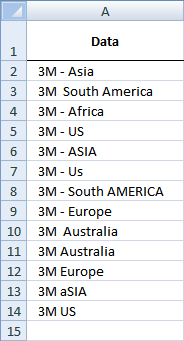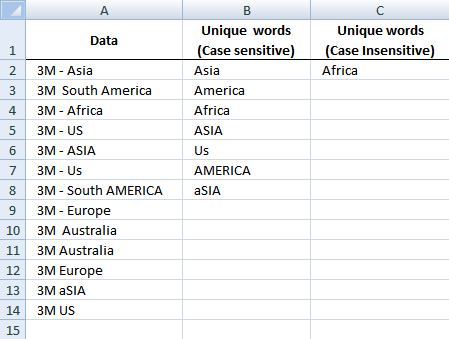Author: Oscar Cronquist Article last updated on January 01, 2019This blog post describes how to create a list of unique words from a cell range. Unique words are all words not having duplicates.

Cell range A2:A14 contains words, see picture.

Rick Rothstein (MVP - Excel) helped me out here with a powerful user defined function (udf).Array formula in cell B2:B23

=UniqueWords(\$A\$2:\$A\$18, TRUE)

This is how you enter an array formula, type the formula in a cell then press and hold CTRL + SHIFT simultaneously, now press Enter once. Release all keys.

The formula bar now shows the formula with a beginning and ending curly bracket telling you that you entered the formula successfully. Don't enter the curly brackets yourself.

Array formula in cell C2:C23

=UniqueWords(\$A\$2:\$A\$18, FALSE)

Select all the cells to be filled, then type the above formula  into the Formula Bar and press CTRL+SHIFT+ENTER

### User defined functionInstructions

1. You can select far more cells to load the formulas in than are required by the list. The empty text string will be displayed for cells not having an entry.
2. You can specify a larger range than the there are filled in cells as the argument to these macros to allow for future entries in the column.
3. You can specify whether the listing is to be case sensitive or not via the optional second argument with the default value being FALSE, meaning duplicated entries with different casing like One, one, ONE, onE, etc.. will all be treated as if they were the same word with the same spelling. If you pass TRUE for that optional second argument, then those words would all be treated as if they were different words.
4. For all the "Case Insensitive" listing, the words are listed in Proper Case (first letter upper case, remaining letters lower case). The reason being if you had One, one and ONE then there is not reason to prefer one version over another, so I solved the problem by using Proper Case throughout.

VBA Code:

```Function UniqueWords(Rng As Range, Optional  CaseSensitive As Boolean) As Variant
Dim X As Long, WordCount As Long,  List As String, Uniques As Variant, Words() As String
List =  WorksheetFunction.Trim(Replace(Join(WorksheetFunction.Transpose(Rng)), Chr(160),  " "))
Words = Split(List)
For X = 0 To  UBound(Words)
If CaseSensitive  Then
If UBound(Split(" " &amp; List &amp; " ",  " " &amp; Words(X) &amp; " ")) = 1  Then
Uniques = Uniques &amp;  Words(X) &amp; " "
List =  Replace(List, Words(X), "")
End  If
Else
If  UBound(Split(" " &amp; UCase(List) &amp; " ", " " &amp; UCase(Words(X)) &amp; "  ")) = 1 Then
Uniques = Uniques  &amp; StrConv(Words(X), vbProperCase) &amp; "  "
List = Replace(List, Words(X),  "")
End If
End  If
Next
Uniques = WorksheetFunction.Trim(Uniques)
Words = Split(Uniques)
If Application.Caller.Count &gt; UBound(Words)  Then
Uniques = Uniques &amp;  Space(Application.Caller.Count - UBound(Words))
End If
UniqueWords = WorksheetFunction.Transpose(Split(Uniques))
End  Function
```

### How to implement user defined function in excel

1. Press Alt-F11 to open visual basic editor
2. Press with left mouse button on Module on the Insert menu
3. Copy and paste the above user defined function
4. Exit visual basic editor
5. Select a sheet
6. Select a cell range
7. Type =UniqueWords(\$A\$2:\$A\$18, TRUE) into formula bar and press CTRL+SHIFT+ENTER

### Get the Excel fileUnique-words-from-a-range.xls

### Get the Excel fileWord-Listing-Code1.xls

Many thanks to Rick Rothstein (Mvp - Excel)!!